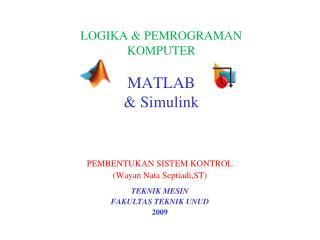# LOGIKA & PEMROGRAMAN KOMPUTER MATLAB & Simulink - PowerPoint PPT PresentationDownload PresentationLOGIKA & PEMROGRAMAN KOMPUTER MATLAB & Simulink

LOGIKA & PEMROGRAMAN KOMPUTER MATLAB & SimulinkDownload Presentation## LOGIKA & PEMROGRAMAN KOMPUTER MATLAB & Simulink

- - - - - - - - - - - - - - - - - - - - - - - - - - - E N D - - - - - - - - - - - - - - - - - - - - - - - - - - -
##### Presentation Transcript

1. LOGIKA & PEMROGRAMAN KOMPUTERMATLAB & Simulink PEMBENTUKAN SISTEM KONTROL (Wayan Nata Septiadi,ST) TEKNIK MESIN FAKULTAS TEKNIK UNUD 2009

2. Some Advanced Features • Creating hierarchical models using subsystems. • Writing S-functions for Customization & Creating new blocks. • Implementation of Conditions & Logics. • Importing CAD models to SimMechanics. • Creating Virtual Worlds & Animations. • Soft Computing in Control. • Real-Time Technology & Hardware in the Loop Simulations.

3. Example: A Control System This example illustrates the modeling of a more complex system, which is the thermodynamics of a house and its temperature control system. This demo also illustrate the usage of subsystems to create hierarchical models.

4. S-Functions • You can use S-Functions to customize and createyour own blocks. • An interesting example is providing custom animations for Simulink block-diagram models.A simple example of a mass-spring sys with an animation S-Function is provided as a demo named dblcart1 in the software documentation. • A general M-file S-function template is also provided in: C:\MATLAB\toolbox\simulink\blocks\sfuntmpl.m which can be used as a guide to write M-file S-functions. For more information see the separate Writing S-Functions PDF guide.

5. Implementation ofConditions &Logics • Simulink: Conditionally Executed Subsystems: enabled/triggered/triggered-enabled subsystems Control Flow Statements & Blocks • StateFlow: For implementation of more complex Flow Control Logics (Event-controlled models)

7. Virtual Reality Toolbox

8. SimMechanics What Is SimMechanics? • SimMechanics is a block diagram modeling environment for the engineering design and simulation of rigid body machines and their motions, using the standard Newtonian dynamics of forces and torques.

9. Physical vs. Mathematical Modeling • Simulink (normal) blocks:represent mathematical operations or operate on signals, • Physical Modeling blocks:represent physical components or relationships directly, Note:Sensors & Actuators are used to connect these two kinds of blocks.

10. SimMechanics Analysis Modes: • Forward Dynamics analysis: integrates applied forces/torques, maintaining imposed constraints, and obtains resulting motions. • Inverse Dynamics analysis: finds the forces/torques necessary to produce user-specified motions in topologically open systems. • Kinematics analysis: finds the forces/torques necessary to produce user-specified motions in topologically closed (loop) systems. • Trimming analysis: searches for steady or equilibrium states of a system.

11. SimMechanics Block Libraries • Bodies Library • Joints Library • Constraints & Drivers Library • Sensors & Actuators Library • Force Elements Library • Utilities Library

12. Essential Steps to Build a Model • Select Ground, Body, and Joint blocks. • Position and connect blocks. • Configure Body blocks. • Configure Joint blocks. • Select, connect, and configure Constraint and Driver blocks. • Select, connect, and configure Actuator and Sensor blocks. • Encapsulate subsystems.

13. Example#1Modeling a Double Pendulum

14. Example#2 • One Degree of Freedom Mass-Spring-Damper System • Solution Methods: MATLAB:Solving the DE with an IVP/ODE solver Control Toolbox:Transfer Fcn, State Space Modeling Simulink:DE-solving, Transfer Fcn, State Space SimMechanics:Physical Modeling

15. Using Body Spring & Damper

16. Using Joint Spring & Damper

17. Using Sensor-Actuator Feedback

18. Assignment 2-D Bounce & Pitch Vehicle Model • Model the suspension system physically using SimMechanics. • Compare the modeling approach with the mathematical one, done previously using Simulink.